Courses

# Chemistry Test - 1

## 45 Questions MCQ Test | Chemistry Test - 1

Description
This mock test of Chemistry Test - 1 for helps you for every entrance exam. This contains 45 Multiple Choice Questions for Chemistry Test - 1 (mcq) to study with solutions a complete question bank. The solved questions answers in this Chemistry Test - 1 quiz give you a good mix of easy questions and tough questions. students definitely take this Chemistry Test - 1 exercise for a better result in the exam. You can find other Chemistry Test - 1 extra questions, long questions & short questions for on EduRev as well by searching above.
QUESTION: 1

Solution:
QUESTION: 2

Solution:
QUESTION: 3

### Decreasing order of size of various hybrid orbitals is

Solution:
QUESTION: 4

Which set of quantum numbers are not applicable to an electron ?

Solution:
QUESTION: 5

The energy of an electron of 2py orbital is

Solution:
QUESTION: 6

The de Broglie wavelength associated with particle is

Solution:
QUESTION: 7

The angular momentum of 3rd orbit is

Solution:
QUESTION: 8

Which one of the following has the highest value of ionic radius ?

Solution:
QUESTION: 9

Non directional orbital is

Solution:
QUESTION: 10

The bond length in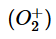, O2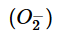and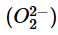follows the order

Solution:
QUESTION: 11

The shape of covalent molecule MX3 is

Solution:
QUESTION: 12

The rate of diffusion of hydrogen is about

Solution:
QUESTION: 13

At STP the order of root mean square speed of molecules H2, N2, O2 and HBr is

Solution:
QUESTION: 14

Two separate bulbs contain gas A and gas B. The density of a gas A is twice as that of B. The molecular mass of A is 1/2 as that of B. If temperature is constant, the ratio of the pressure of A and B is

Solution:
QUESTION: 15

A quantity of hydrogen gas occupies a volume of 30.0 mL at a certain temperature and pressure. What volume of half of this mass of hydrogen occupy at triple the absolute temperature if the pressure were one-ninth that of original gas ?

Solution:
QUESTION: 16

Oxygen and cyclopropane at partial pressure of 570 torr and 170 torr respectively are mixed in a gas cylinder. The ratio of the number of molecules of cyclopropane to the number of moles of oxygen is

Solution:
QUESTION: 17

x L N2 gas at STP contains 3 x 1022 molecules. The number of molecules in x L ozone at STP will be

Solution:
QUESTION: 18

4.0 g of argon has pressure P at temperature T K in a vessel. On keeping the sample at 500 higher temperature, 0.8 g gas was given out to maintain the pressure P. The original temperature was

Solution:
QUESTION: 19

What is the mass per cent of carbon in carbon dioxide ?

Solution:
QUESTION: 20

If the pressure of N2 /H2 mixture in a closed vessel is 100 atmosphere and 20% of the mixture then reacts, the pressure at the same temperature would be

Solution:
QUESTION: 21

1.35 g of pure Ca metal was quantitatively converted into 1.88 g of pure CaO. What is atomic weight of Ca?

Solution:
QUESTION: 22

Paramagnetism of oxygen is explained on the basis of the electronic configuration of

Solution:
QUESTION: 23

An element A is tetravalent and another element B is divalent. The formula of the compound formed from these elements will be

Solution:
QUESTION: 24

For three different gases values of van der Waals’ constant ‘a’ and ‘b’ are given. What is the correct order of liquefication of gases ?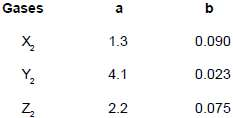Solution:
QUESTION: 25

Number of electrons in 1.8 mL of H2O is

Solution:
QUESTION: 26

Assuming fully decomposed, the volume of CO2 released at STP, on heating 9.85 g of BaCO3 (at. wt. of Ba = 137) will be

Solution:
QUESTION: 27

Find the rms speed of argon molecule at 270C (Molecular weight of argon = 40 gm/mol)

Solution:
QUESTION: 28

Molarity of H2SO4 (density 1.8 g/mL) is 18 M. The molality of this H2SO4 will be

Solution:
QUESTION: 29

Number of oxalic acid molecules in 100 mL of 0.02 N oxalic acid are

Solution:
QUESTION: 30

Number of ions present in 2.0 litre of a solution of 0.8 M K4[Fe(CN6)]  is

Solution:
QUESTION: 31

The hybridization of carbon atoms in C-C single bond of CH ≡C-CH=CH2 is

Solution:
QUESTION: 32

The hybrid states of carbon in diamond, graphite and acetylene are respectively

Solution:
QUESTION: 33

In sp3d3 hybridization, which d-orbitals are involved?

Solution:
QUESTION: 34

Which of the following pairs is isoelectronic ?

Solution:
QUESTION: 35

How many unpaired electrons are present in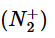?

Solution:
QUESTION: 36

Which is not true in case of an ideal gas ?

Solution:
QUESTION: 37

The correct hybridization state of sulphur atom in SF2, SF4 and SF6 molecules is respectively

Solution:
QUESTION: 38

An atom has 2 electrons in K shell, 8 electrons in L shell and 6 electrons in M shell. the number of s electrons present in that element is

Solution:
QUESTION: 39

The correct order of increasing C - O bond lengths in CO,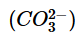and CO2 is

Solution:
QUESTION: 40

The number of unpaired electrons in Cr3+ is

Solution:
QUESTION: 41

The correct order for electronegativty of halogens is

Solution:
QUESTION: 42

Which of the following is wrong statement ?

Solution:
QUESTION: 43

Which of the following spectral series of hydrogen atom lies in the far infrared region of electromagnetic radiation?

Solution:
QUESTION: 44

Alkaline earth metals

Solution:
QUESTION: 45

The halogens

Solution:

Track your progress, build streaks, highlight & save important lessons and more!

### Similar Content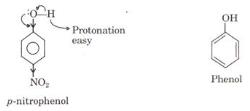### Related tests